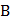# If two vertices of a triangle areand, orthocentre lies at the origin and centroid on the line, then the third vertex lies at a) (7, 4) b) (8, 14) c) (12, 21) d) None of these

## Question ID - 52929 :- If two vertices of a triangle areand, orthocentre lies at the origin and centroid on the line, then the third vertex lies at a) (7, 4) b) (8, 14) c) (12, 21) d) None of these

3537

(d)

Givenis the orthocentre. Letbe the third vertex,andthe other two vertices. Then the slope of the line throughandis, while the lines throughandhas the slope. By the property of the orthocenter, these two lines must be perpendicular, so we have(i)

Also,(ii)

Which is not satisfied by the points given inorNext Question :
 Pointsandare in the first quadrant; point '' is the origin. If the slope ofis 1, slope ofis 7 and, then the slope ofis a)b)c)d)